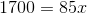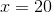Example Questions

Example Question #1081 : Sat Mathematics

An artist's new album sold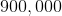copies on its release date. If the U.S. makes upof these sales, how many copies were sold on that day in the U.S.?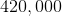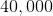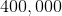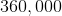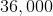Explanation:

Let's set up a proportion to solve this problem, whererepresents the number of copies sold in the U.S. Remember that we can expressas a fraction.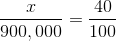Now, we can solve for the unknown by cross-multiplying.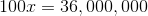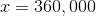Example Question #1082 : Sat Mathematics

Jane called one thousand times to tell you she's sorry. If you saw she was calling and let your phone go to voicemail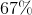of the time, how many voicemails would you have received if she left one each time?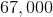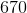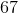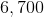Explanation:

To solve this problem, we can set up a proportion. Remember we can express percentages as fractions. Letrepresent the number of voicemails.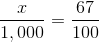Now, we can cross-multiply and solve for the unknown.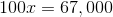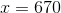Example Question #1083 : Sat Mathematicsis what percentage of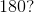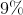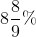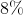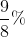Cannot be determinedExplanation:

To solve this we need to set up a proportion.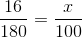Now we cross multiply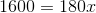Divide by 180.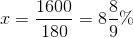Example Question #1 : Whole And Part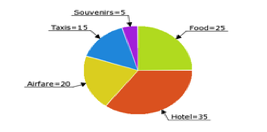David's trip expenses are pictured in the above pie chart (numbers = % of his total expenses). If he spent $75 on taxis, how much did he spend on hotel and souvenirs combined? Possible Answers:$175

$250$225

$200$40

$200 Explanation: David spent$75 on taxis, which were 15% of his total expenses on the trip. He therefore spent 75(100/15) = $500 on the trip altogether. The hotel and souvenirs make up 35% + 5% = 40% of his total expenses. 40% of 500 is$200.

Example Question #2 : Whole And Part

30% of what number is 20?

Solve to the nearest hundredth.

0.67

1.5

66.67

150%

66.67

Explanation:

This is a very basic form percentage question. This can be rewritten:

0.3 * x = 20

(Remember, the word "of" in a word problem indicates multiplication, while the word "is" indicates an equals sign).

Solve for x: x = 20 / 0.3 = 66.67

A toy is on sale for 43% off. Its sale price is $21.37. What is the full price? Possible Answers: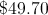None of the available answers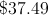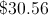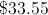Correct answer:Explanation: An algebraic expression for this item is: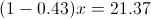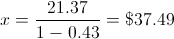Example Question #4 : Whole And Part Twenty-six students planned to contribute an equal amount to purchase a gift for their teacher. After 18 students had paid, they had collected$76.50. What is the total price of the gift?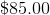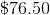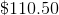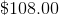Explanation:

If $76.50 had been collected after 18 students had paid, we can determine how much each student contributed:$76.50/18 = $4.25 per student Now we can multiply this by the total number of students (26) to get the full price of the gift: 26 x$4.25 = \$110.50

Example Question #5 : Whole And Part

If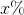ofis, then what isof?

50

100

200

20

10

50

Explanation:

The first part of the problem tells us that x% of 20 is 50. We can model x% as x/100 or 0.01x. To find x% of 20, we can multiply 0.01x and 20. In other words, we can write the following equation:

(0.01x)(20) = 50

Divide both sides by 20.

0.01x = 2.5

Divide both sides by 0.01.

x = 250.

The question then asks us to find 20% of x. We can represent 20% as 0.2, and we know that x is 250. Therefore,

20% of 250 = 0.2(250) = 50.

Example Question #6 : Whole And Part

Ifof a number is, what isof the number?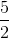Explanation:

Let x be the number in question.

Then 2/3 * x = 18.

x = 18 * 3/2 = 27

Now find 1/9 of 27:

1/9 * 27 = 3

Example Question #41 : Percentage

17 is 85% of what number?Explanation:

In this case, 17 is part of a whole x. We are also given that 17 is 85% of x.

With this in mind, we can set up the following proportion.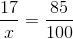To solve for x, cross multiply.